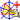Go to content
The vector equation of the blue line through AB is given by: r = a + tb

## Software/Applets used on this pageUses the CabriJava interactive geometry applet. You can download the file by double-clicking on the display and then selecting the option at bottom right. You can then load it into your copy of Cabri.

## Glossary

### equation

A statement that two mathematical expressions are equal.

### vector

A mathematical object with magnitude and direction.

Full Glossary List

## This question appears in the following syllabi:

SyllabusModuleSectionTopicExam Year
AQA A-Level (UK - Pre-2017)C4Vectors2D Vector geometry-
CBSE XII (India)Vectors and 3-D GeometryVectorsVectors and scalars, magnitude and direction of a vector-
CCEA A-Level (NI)C4Vectors2D Vector geometry-
CIE A-Level (UK)P1Vectors2D Vector geometry-
Edexcel A-Level (UK - Pre-2017)C4Vectors2D Vector geometry-
Edexcel AS Further Maths 2017Core Pure MathsVectorsVector Algebra-
Edexcel AS/A2 Further Maths 2017Core Pure MathsVectorsVector Algebra-
I.B. Higher Level4Vectors2D Vector geometry-
I.B. Standard Level4Vectors2D Vector geometry-
Methods (UK)M4Vectors2D Vector geometry-
OCR A-Level (UK - Pre-2017)C4Vectors2D Vector geometry-
OCR AS Further Maths 2017Pure CoreVectorsVector Algebra-
OCR MEI AS Further Maths 2017Core Pure AVectors and 3-D SpaceVector Algebra-
OCR-MEI A-Level (UK - Pre-2017)C4Vectors2D Vector geometry-
Pre-Calculus (US)E1Vectors2D Vector geometry-
Pre-U A-Level (UK)6Vectors2D Vector geometry-
Scottish (Highers + Advanced)HM3Vectors2D Vector geometry-
Scottish HighersM3Vectors2D Vector geometry-
Universal (all site questions)VVectors2D Vector geometry-
WJEC A-Level (Wales)C4Vectors2D Vector geometry-